JMSLTM Numerical Library 7.2.0
com.imsl.stat

Class Dissimilarities

• All Implemented Interfaces:
Serializable, Cloneable

public class Dissimilarities
extends Object
implements Serializable, Cloneable
Computes a matrix of dissimilarities (or similarities) between the columns (or rows) of a matrix.

Class Dissimilarities computes an upper triangular matrix (excluding the diagonal) of dissimilarities (or similarities) between the columns (or rows) of a matrix. Nine different distance measures can be computed. For the first three measures, three different scaling options can be employed. The distance matrix computed is generally used as input to clustering or multidimensional scaling functions.

The following discussion assumes that the distance measure is being computed between the columns of the matrix. If distances between the rows of the matrix are desired, use row = true in the setRow method.

The distance method and scaling option used by Dissimilarities can be set via methods setDistanceMethod and setScalingOption, respectively. For distance methods L2_NORM, L1_NORM, or INFINITY_NORM, each row of x is first scaled according to the value specified by the setScalingOption method. The scaling parameters are obtained from the values in the row scaled as either the standard deviation of the row or the row range; the standard deviation is computed from the unbiased estimate of the variance. If no scaling is performed, the parameters in the following discussion are all 1.0 (see setScalingOption). Once the scaling value (if any) has been computed, the distance between column i and column j is computed via the difference vector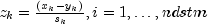, where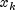denotes the k-th element in the i-th column,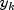denotes the corresponding element in the j-th column, and ndstm is the number of rows if differencing columns and the number of columns if differencing rows. For given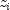, the distance methods that allow scaling are defined as:

distanceMethod Metric
L2_NORMEuclidean distance (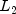norm)
L1_NORMSum of the absolute differences (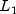norm)
INFINITY_NORMMaximum difference (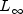norm)

The following distance measures do not allow for scaling.

distanceMethod Metric
MAHALANOBISMahalanobis distance
ABS_COSINEAbsolute value of the cosine of the angle between the vectors
ANGLE_IN_RADIANSAngle in radians (0,) between the lines through the origin defined by the vectors
CORRELATION_COEFFICIENTCorrelation coefficient
ABS_CORRELATION_COEFFICIENTAbsolute value of the correlation coefficient
EXACT_MATCHESNumber of exact matches, where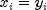.

For the Mahalanobis distance, any variable used in computing the distance measure that is (numerically) linearly dependent upon the previous variables in the indexArray vector from the setIndex method is omitted from the distance measure.

Example 1, Example 2, Serialized Form
• Field Summary

Fields
Modifier and Type Field and Description
static int ABS_CORRELATION_COEFFICIENT
Indicates the absolute value of the correlation coefficient distance method.
static int ABS_COSINE
Indicates the absolute value of the cosine of the angle between the vectors distance method.
Indicates the angle in radians (0,) between the lines through the origin defined by the vectors distance method.
static int CORRELATION_COEFFICIENT
Indicates the correlation coefficient distance method.
static int EXACT_MATCHES
Indicates the number of exact matches distance method.
static int INFINITY_NORM
Indicates the maximum difference (norm) distance method.
static int L1_NORM
Indicates the sum of the absolute differences (norm) distance method.
static int L2_NORM
Indicates the Euclidean distance method (norm).
static int MAHALANOBIS
Indicates the Mahalanobis distance method.
static int NO_SCALING
Indicates no scaling.
static int RANGE
Indicates scaling by the range.
static int STD_DEV
Indicates scaling by the standard deviation.
• Constructor Summary

Constructors
Constructor and Description
Dissimilarities(double[][] x)
Constructor for Dissimilarities.
• Method Summary

Methods
Modifier and Type Method and Description
void compute()
Computes a matrix of dissimilarities (or similarities) between the columns (or rows) of a matrix.
double[][] getDistanceMatrix()
Returns the distance matrix.
int getDistanceMethod()
Returns the method used in computing the dissimilarities or similarities.
int[] getIndex()
Returns the indices of the rows (columns) used in computing the distance measure.
boolean getRow()
Returns a boolean indicating whether distances are computed between rows or columns of x.
int getScalingOption()
Returns the scaling option.
void setDistanceMethod(int distanceMethod)
Sets the method to be used in computing the dissimilarities or similarities.
void setIndex(int[] indexArray)
Sets the indices of the rows (columns).
void setRow(boolean row)
Identifies whether distances are computed between rows or columns of x.
void setScalingOption(int distanceScale)
Sets the scaling option used if the L2_NORM, L1_NORM, or INFINITY_NORM distance methods are specified.
• Field Detail

• ABS_CORRELATION_COEFFICIENT

public static final int ABS_CORRELATION_COEFFICIENT
Indicates the absolute value of the correlation coefficient distance method.
Constant Field Values
• ABS_COSINE

public static final int ABS_COSINE
Indicates the absolute value of the cosine of the angle between the vectors distance method.
Constant Field Values

Indicates the angle in radians (0,) between the lines through the origin defined by the vectors distance method.
Constant Field Values
• CORRELATION_COEFFICIENT

public static final int CORRELATION_COEFFICIENT
Indicates the correlation coefficient distance method.
Constant Field Values
• EXACT_MATCHES

public static final int EXACT_MATCHES
Indicates the number of exact matches distance method.
Constant Field Values
• INFINITY_NORM

public static final int INFINITY_NORM
Indicates the maximum difference (norm) distance method.
Constant Field Values
• L1_NORM

public static final int L1_NORM
Indicates the sum of the absolute differences (norm) distance method.
Constant Field Values
• L2_NORM

public static final int L2_NORM
Indicates the Euclidean distance method (norm).
Constant Field Values
• MAHALANOBIS

public static final int MAHALANOBIS
Indicates the Mahalanobis distance method.
Constant Field Values
• NO_SCALING

public static final int NO_SCALING
Indicates no scaling.
Constant Field Values
• RANGE

public static final int RANGE
Indicates scaling by the range.
Constant Field Values
• STD_DEV

public static final int STD_DEV
Indicates scaling by the standard deviation.
Constant Field Values
• Constructor Detail

• Dissimilarities

public Dissimilarities(double[][] x)
Constructor for Dissimilarities.
Parameters:
x - A double matrix containing the data input matrix.
• Method Detail

• getDistanceMatrix

public final double[][] getDistanceMatrix()
Returns the distance matrix.
Returns:
A double matrix containing the distance matrix.
• getDistanceMethod

public int getDistanceMethod()
Returns the method used in computing the dissimilarities or similarities.
• getIndex

public int[] getIndex()
Returns the indices of the rows (columns) used in computing the distance measure.
• getRow

public boolean getRow()
Returns a boolean indicating whether distances are computed between rows or columns of x.
• getScalingOption

public int getScalingOption()
Returns the scaling option.
• setDistanceMethod

public void setDistanceMethod(int distanceMethod)
Sets the method to be used in computing the dissimilarities or similarities.
Parameters:
distanceMethod - An int identifying the method to be used in computing the dissimilarities or similarities. Acceptable values of distanceMethod are:

distanceMethod Metric
L2_NORMEuclidean distance (norm)
L1_NORMSum of the absolute differences (norm)
INFINITY_NORMMaximum difference (norm)
MAHALANOBISMahalanobis distance
ABS_COSINEAbsolute value of the cosine of the angle between the vectors
ANGLE_IN_RADIANSAngle in radians (0,) between the lines through the origin defined by the vectors
CORRELATION_COEFFICIENTCorrelation coefficient
ABS_CORRELATION_COEFFICIENTAbsolute value of the correlation coefficient
EXACT_MATCHESNumber of exact matches, where.

See class description for more details. By default, distanceMethod = L2_NORM.
• setIndex

public void setIndex(int[] indexArray)
Sets the indices of the rows (columns).
Parameters:
indexArray - An int array containing the indices of the columns (rows if row = false) to be used in computing the distance measure. By default, if row = true, indexArray = 0, 1, ..., x.length-1. If row = false, indexArray = 0, 1, ..., x.length-1, see setRow.
• setRow

public void setRow(boolean row)
Identifies whether distances are computed between rows or columns of x.
Parameters:
row - A boolean identifying whether distances are computed between rows or columns of x. If row = true, distances are computed between the rows of x. Otherwise, distances between the columns of x are computed. By default, row = true.
• setScalingOption

public void setScalingOption(int distanceScale)
Sets the scaling option used if the L2_NORM, L1_NORM, or INFINITY_NORM distance methods are specified. See setDistanceMethod.
Parameters:
distanceScale - An int containing the scaling option. By default, distanceScale = NO_SCALING.

distanceScale Method
NO_SCALINGNo scaling is performed.
STD_DEV If setRow(false), scale each column by the standard deviation of the column.
If setRow(true), scale each row by the standard deviation of the row.
RANGE If setRow(false), scale each column by the range of the column.
If setRow(true), scale each row by the range of the row.

JMSLTM Numerical Library 7.2.0